Core Assessments Core 1 This Friday 54 Core

• Slides: 18Core Assessments • Core #1: This Friday (5/4) • Core #2: Tuesday, 5/8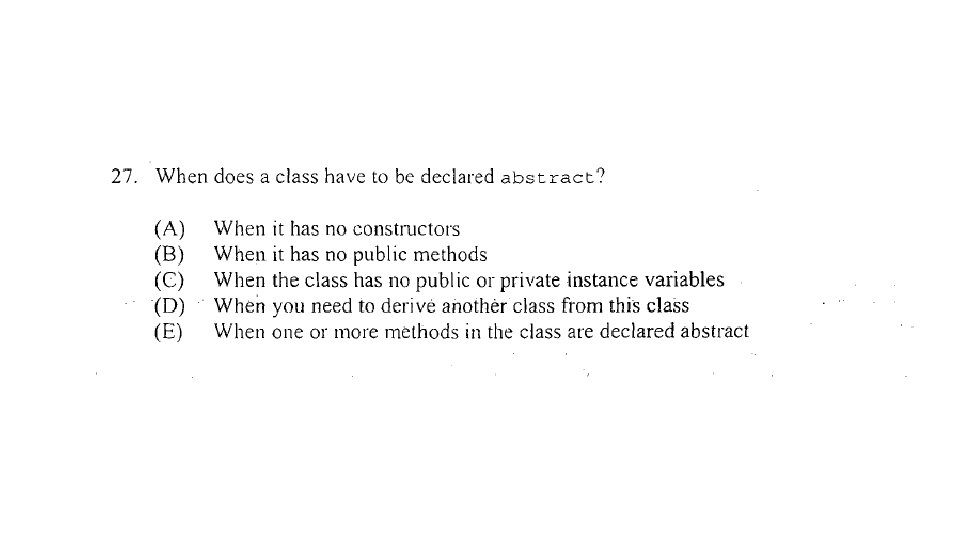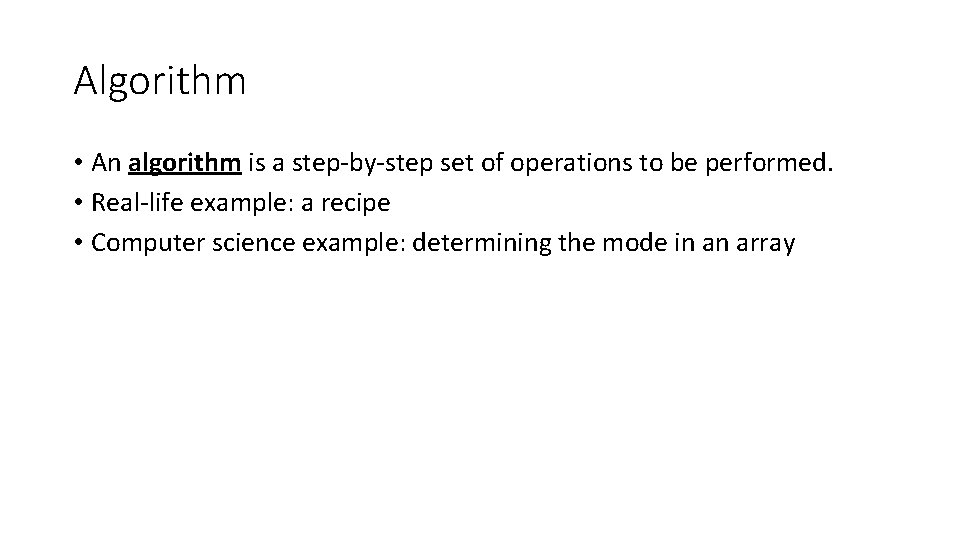Algorithm • An algorithm is a step-by-step set of operations to be performed. • Real-life example: a recipe • Computer science example: determining the mode in an array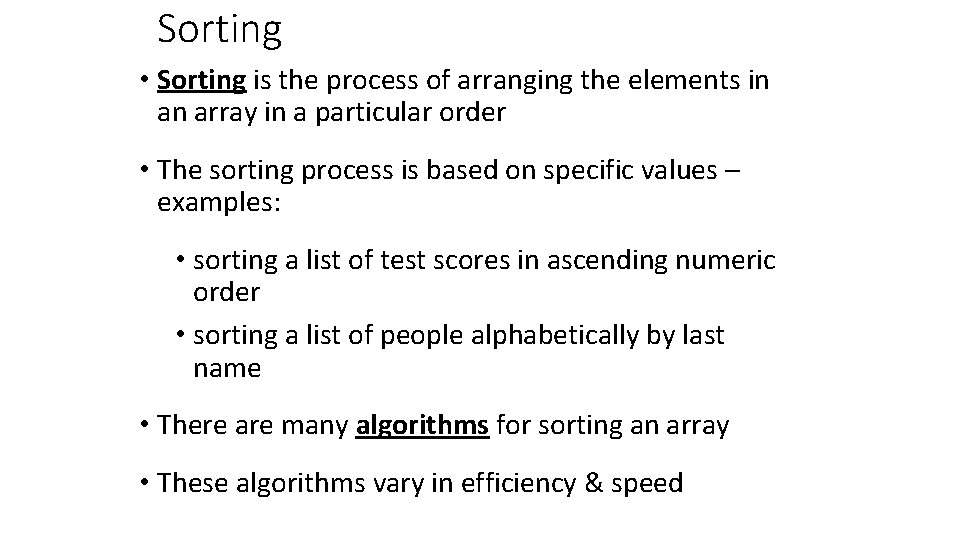Sorting • Sorting is the process of arranging the elements in an array in a particular order • The sorting process is based on specific values – examples: • sorting a list of test scores in ascending numeric order • sorting a list of people alphabetically by last name • There are many algorithms for sorting an array • These algorithms vary in efficiency & speed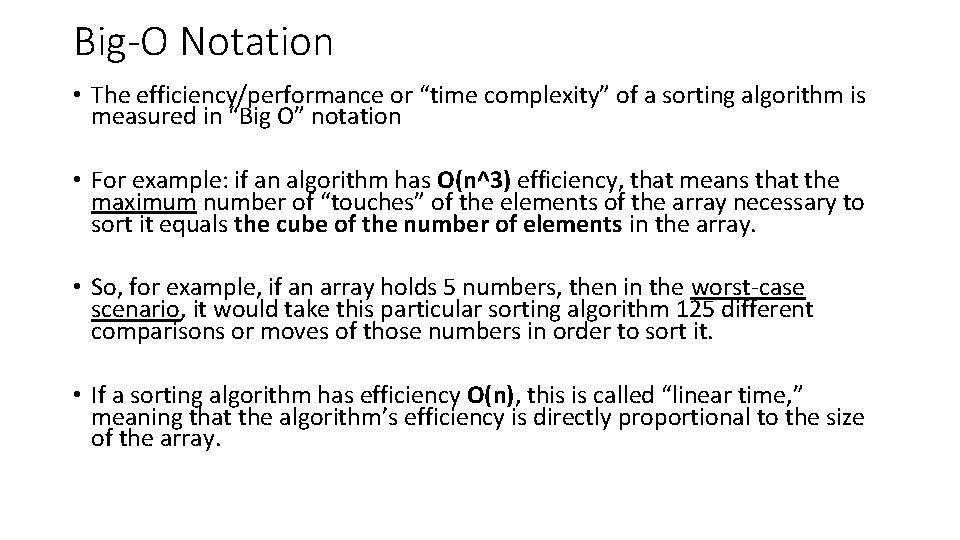Big-O Notation • The efficiency/performance or “time complexity” of a sorting algorithm is measured in “Big O” notation • For example: if an algorithm has O(n^3) efficiency, that means that the maximum number of “touches” of the elements of the array necessary to sort it equals the cube of the number of elements in the array. • So, for example, if an array holds 5 numbers, then in the worst-case scenario, it would take this particular sorting algorithm 125 different comparisons or moves of those numbers in order to sort it. • If a sorting algorithm has efficiency O(n), this is called “linear time, ” meaning that the algorithm’s efficiency is directly proportional to the size of the array.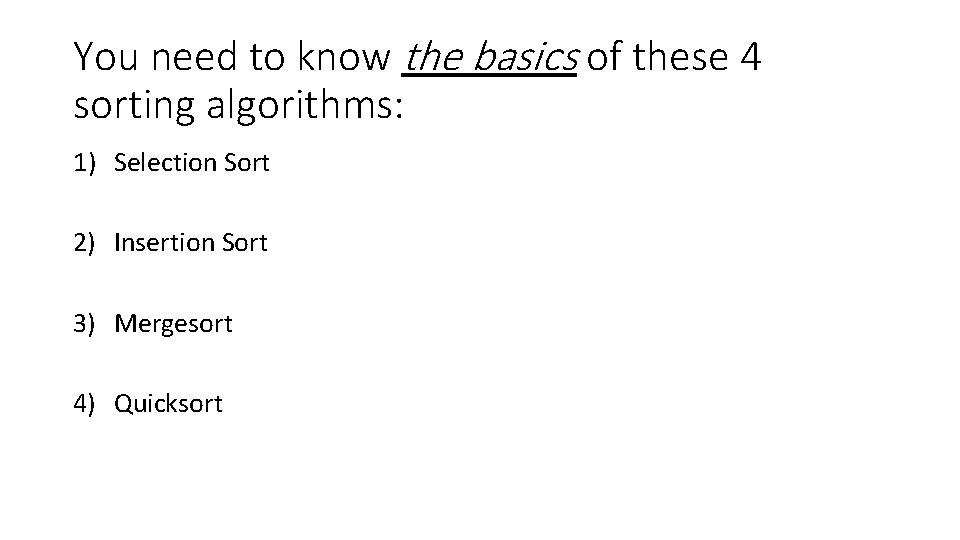You need to know the basics of these 4 sorting algorithms: 1) Selection Sort 2) Insertion Sort 3) Mergesort 4) QuicksortSelection Sort 1. 2. 3. 4. 5. find the smallest element in the array swap it with the first element find the next-smallest element in the array swap it with the second element repeat until all values are in their proper places Efficiency: O(n^2) • Inefficient for large listsSelection Sort example: original array: 3 9 6 1 2 smallest 1 1 9 2 2 2 6 6 3 3 6 6 2 9 9 9 is is 1: 2: 3: 6:Insertion Sort 1) pick an item and insert it into its proper place in a sorted sublist 2) repeat until all items have been inserted Efficiency: O(n) (best-case scenario) or O(n^2) (worst-case scenario) An example: original: insert 3: insert 6: insert 1: insert 2: 9 3 3 1 1 3 9 6 3 2 6 6 9 6 3 1 1 1 9 6 2 2 9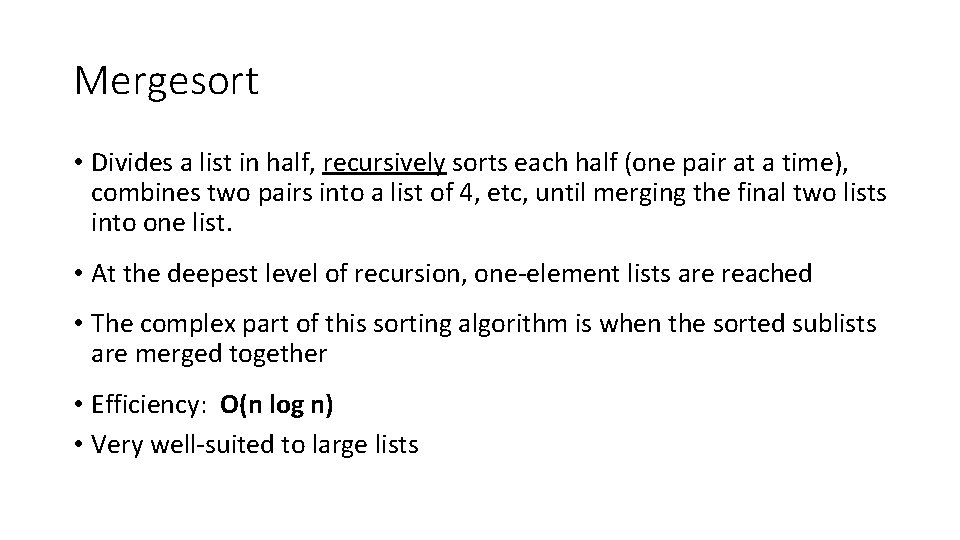Mergesort • Divides a list in half, recursively sorts each half (one pair at a time), combines two pairs into a list of 4, etc, until merging the final two lists into one list. • At the deepest level of recursion, one-element lists are reached • The complex part of this sorting algorithm is when the sorted sublists are merged together • Efficiency: O(n log n) • Very well-suited to large lists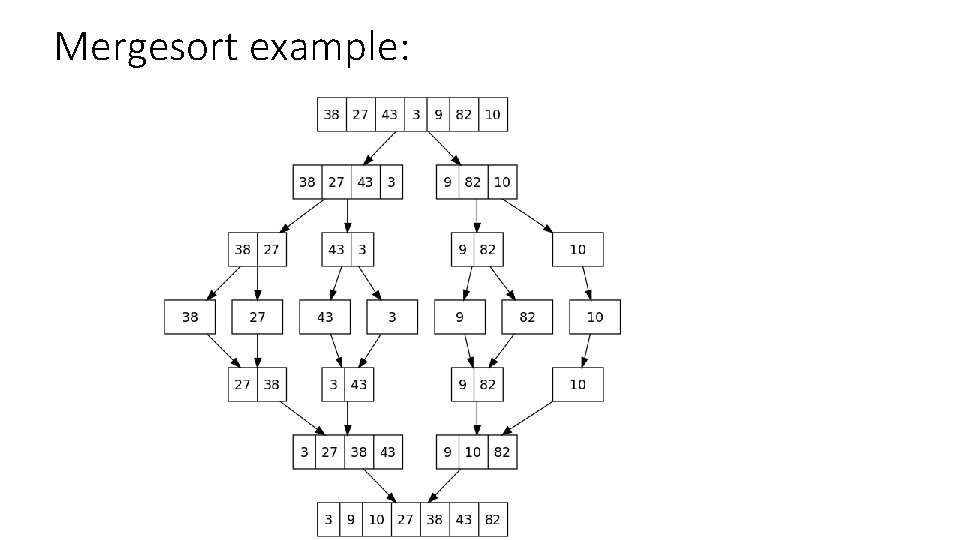Mergesort example:Quicksort • Chooses a pivot value, then partitions the list into two sublists, (one list contains everything smaller than the pivot, the other contains everything larger), then recursively sorts each sublist • Unlike a merge sort, a quick sort does most of its work when it divides the list • It doesn’t need to combine sublists after the recursive steps; the list is already sorted at that point • Also, unlike a merge sort, the 2 sublists do not have to be the same size • One of the more popular sorting techniques • Efficiency: O(n log n) (best-case scenario) or O(n^2) (worst-case scenario)Quicksort example: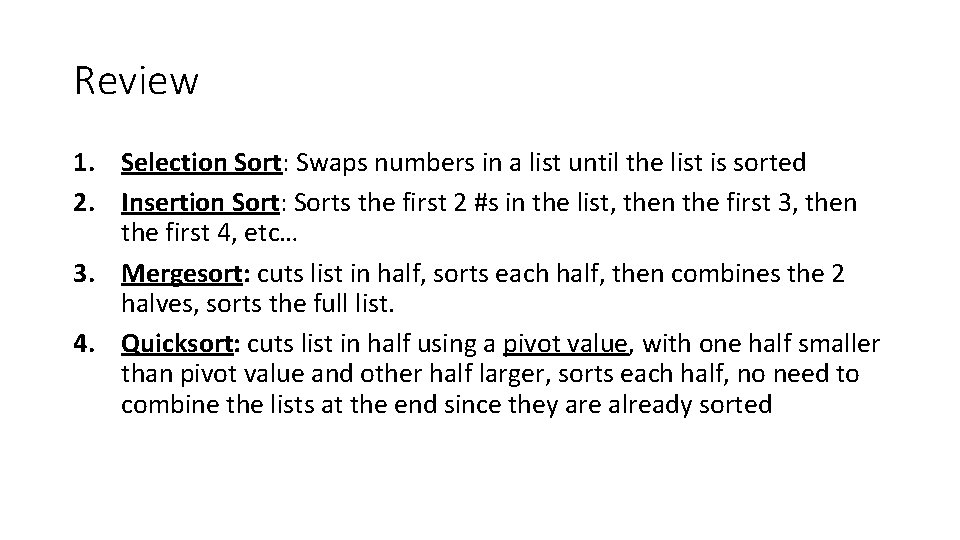Review 1. Selection Sort: Swaps numbers in a list until the list is sorted 2. Insertion Sort: Sorts the first 2 #s in the list, then the first 3, then the first 4, etc… 3. Mergesort: cuts list in half, sorts each half, then combines the 2 halves, sorts the full list. 4. Quicksort: cuts list in half using a pivot value, with one half smaller than pivot value and other half larger, sorts each half, no need to combine the lists at the end since they are already sortedAssignments 1) Open “Sorting Practice Qs” and complete. Save your answers. 2) In Word or in JCreator, complete 2017 FR in the Exam Resources folder. Email me your answers when done. Complete for HW if necessary.